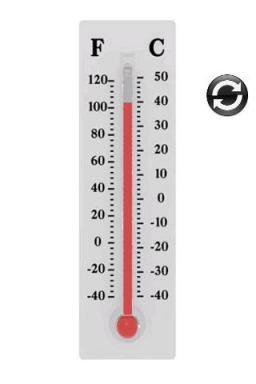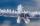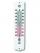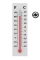# The lowest

The lowest temperature on Thursday was 20°C. The lowest temperature on Saturday was 12°C. What was the difference between the lowest temperatures?

D =  -8 °C

### Step-by-step explanation:

$D=12-20=-8\text{°C}$Did you find an error or inaccuracy? Feel free to write us. Thank you!## Related math problems and questions:

• Difference of temperaturesThe temperature on Saturday was –2°C. The temperature on Sunday was 8°C. Write down the difference between these two temperatures.
• Difference in temperaturesThe temperature in the number line show minus 15 degree celsius and 8 degree celsius. What is the difference between the temperatures?
• The temp rangeWhat is the range in temperatures if the high temperatures is 67 degrees Celsius and the low temperature is -25 degrees Celsius?
• WeatherWeather. At 5:00 am, the temperature was 28°C, and at noontime, it rose by 9°C. By 4:00 pm, it dropped 3°C, and by nighttime, it was 4°C less. What is the room temperature this time?
• Temperature difference 2The temperature in London on new year’s day is -2 degree Celsius. The temperature in Moscow on the same day is -14 degree Celsius, what is the temperature difference between the two cities?
• The freezer1. The temperature inside a freezer is minus 23 degree Celsius. The temperature falls by a further 12 degree degree Celsius. What is the new temperature? 2. What is the difference between temperatures of 12 degree Celsius and 210 degree Celsius?
• Temperature differenceLibya's highest temperature was recorded at 58 degrees Celsius, and the lowest was recorded at -88 degrees Celsius. What is the temperature difference?
• Change in temperatureStarting temperature is 21°C, the highest temperature is 32°C. What is the change in temperature?
• Temperature 2Sunday's high temperature was 3 degrees higher than Saturday's. On Monday, the temperature fell 5 degrees, then rose 7 degrees on Tuesday and 4 more on Wednesday. Then it fell 17 degrees to a record low of 31 on Thursday. What was the temperature on Satur
• OpheliaOphelia recorded the temperature of a cold store every two hours. 1. At 6 am, it was -4°C, and at 8 am, it was -1°C. By how much did the temperature rise? 2. The temperature went up by 5°C in the next two hours. .What was the temperature at 10 am?
• 8 degrees8 degrees Celsius it cools down by 9 degrees Celsius. What is the resultant temperature?
• Mixing Celsius and FahrenheitAdd up three temperatures: 5°F +6°F +0°C
• Celsius degreesThe temperature on Monday was 5 celsius. The temperature on Thursday was 7 degrees less than the temperature on Monday. What was the temperature on Thursday?
• Daily temperatureThe average of daily temperature measurements in one week every day at the same hour was -2.8 °C. We measured all temperatures on different days are different. The highest daily maximum temperature was 2.4 °C, the lowest -6 °C. Determine the options that
• TemperaturesTemperatures in degrees Fahrenheit and Celsius are related by the rule F = 1.8C + 32. What is the temperature in degrees Celsius if it is 50°F?
• Celsius 25 degrees Celsius at midday dropped 12°Celsius degree by evening. What is the temperature?
• LaboratoryMang Elio went to his laboratory at 7:00am And recorded that the temperature was 11.07°C. At lunchtime, the temperature rose by 27.9°C but dropped by 8.05°C at 5:00 PM. What was the temperature at 5:00 PM?